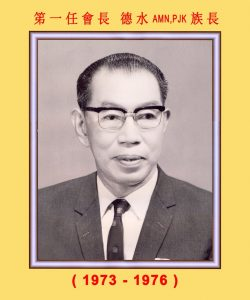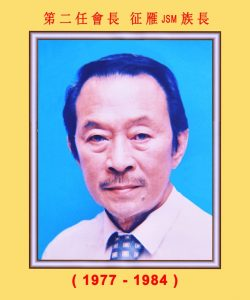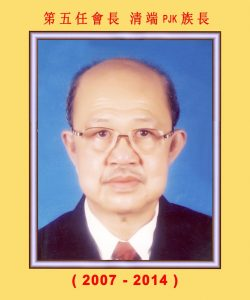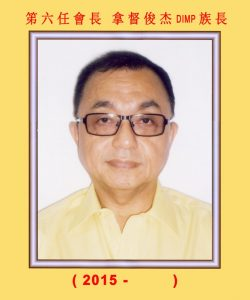hong-pong-family-teaching.pdf : 点击下载

<<<<<<<<<<<<<<<<<<<<<<<<<<<<<<<<<<<<<<<<<<<<<<<<<<<<<<<<<<<<<<<<<<<<<

关怀            ： 陈王美甘

陈黄鹙荭     陈洪瑞元

陈黄鹙荭     陈许秀萍      陈林梅花    陈许幸芬  美叶 丽冰

<<<<<<<<<<<<<<<<<<<<<<<<<<<<<<<<<<<<<<<<<<<<<<<<<<<<<<<<<<<<<<<<<<<<<

华民          再春          梅成

拿督添良   祯森          金基        金振       俊淮      海兴      锦安

### <<<<<<<<<<<<<<<<<<<<<<<<<<<<<<<<<<<<<<<<<<<<<<<<<• ### 筹置会所

1968年族亲大会亦通过成立筹募建会基金小组，成员包括德水、成普、松林、再博、清枝五人，募捐小组耗费两年时间，南下北上，向全马各地族亲劝捐，成绩斐然，兹将捐献者芳名列下：成普、英锦各捐伍仟元，溪水、松林、乔梓合捐伍仟元，志鹏、绍骞各捐叁仟元，德水、德森昆仲合捐叁仟元，金杯、宝琛昆仲合捐叁仟元，庆云、初荣、再博、朝进、添来、克塔合捐壹仟元，克化、克练、克珠、城兴、增元、清河、三发各捐壹仟元，（捐伍佰元以下者为数甚众，恕未克尽录）共计筹得伍万余元，变卖鸿榜胶园得二万一千元，合计七万三千余元。 1970年9月21日，经筹委会议决：以六万元定购巴生惹兰伊斯丹那门牌20号正在建筑中之三层楼大厦，并于1972年3月16日正式迁入办公，成为本会永久会所。

• ### 回乡寻根

（一）建设鸿安大桥（1976年），海外筹集资金马币七万多元，

（二）修建家乡南山公嗣堂，耗费马币五、六万元，1987年完成，

（三）扩建鸿安小学，总捐献马币二十多万元，1994年完成，其他小工程不赘述。 行善本无肤色之分，更无疆界之限，族贤们的苦心与热忱，应受吾人敬仰及学习！

（注：本文资料取自本会成立十二周年及廿五周年纪念特刊，如有错漏，敬请包涵！）